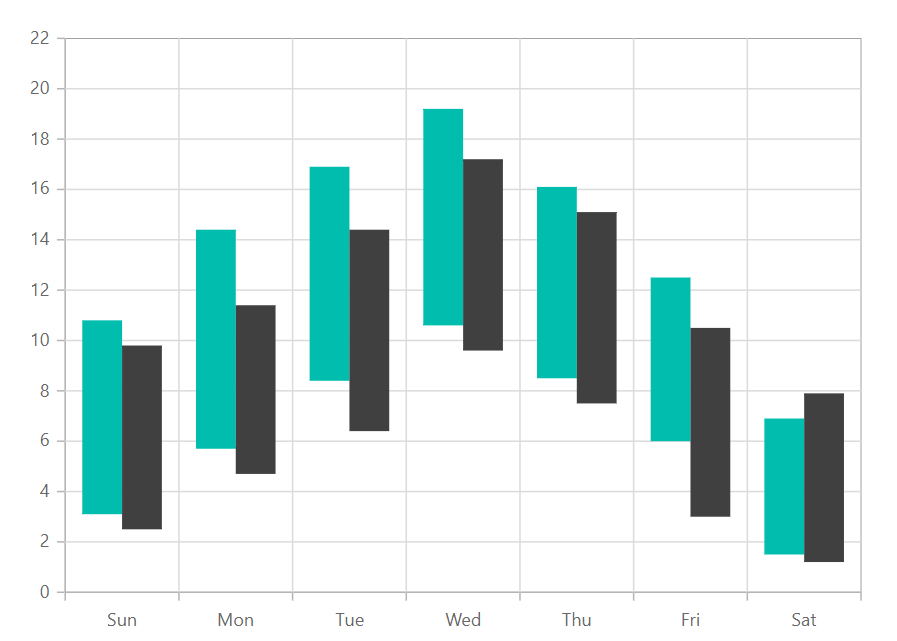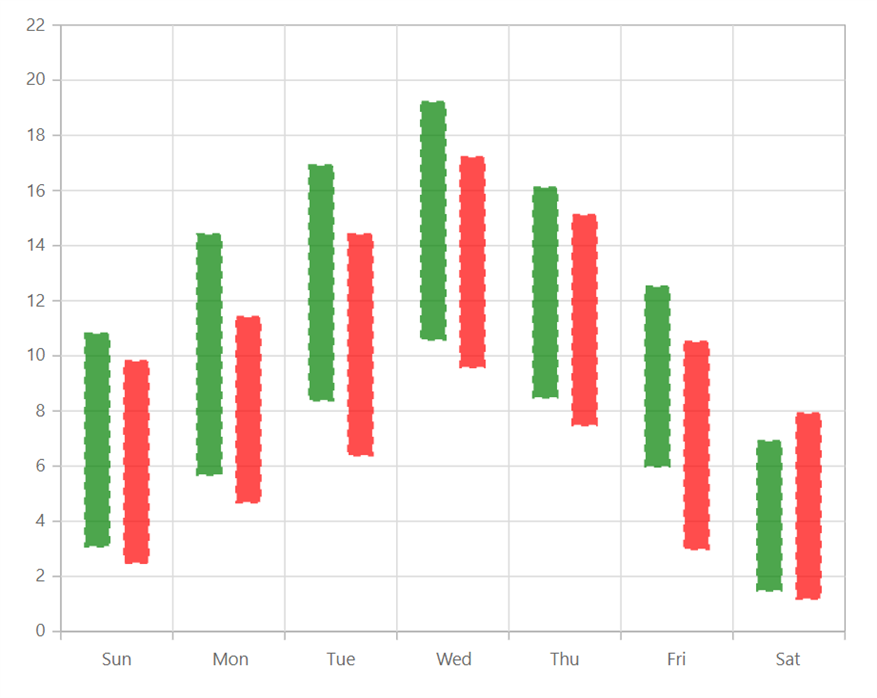# Range Column in Blazor Charts Component

17 Nov 20235 minutes to read

## Range Column

Range Column Chart shows variation in the data values for a given time using vertical bars. To render a Range Column Chart, set the series Type as RangeColumn.

``````@using Syncfusion.Blazor.Charts

<SfChart>
<ChartPrimaryXAxis ValueType="Syncfusion.Blazor.Charts.ValueType.Category"></ChartPrimaryXAxis>

<ChartSeriesCollection>
<ChartSeries DataSource="@WeatherReport1" XName="X" High="High" Low="Low" Width="2" Type="ChartSeriesType.RangeColumn">
</ChartSeries>
<ChartSeries DataSource="@WeatherReport2" XName="X" High="High" Low="Low" Width="2" Type="ChartSeriesType.RangeColumn">
</ChartSeries>
</ChartSeriesCollection>
</SfChart>

@code{
public class ChartData
{
public string X { get; set; }
public double Low { get; set; }
public double High { get; set; }
}

public List<ChartData> WeatherReport1 = new List<ChartData>
{
new ChartData { X= "Sun", Low= 3.1, High= 10.8 },
new ChartData { X= "Mon", Low= 5.7, High= 14.4 },
new ChartData { X= "Tue", Low= 8.4, High= 16.9 },
new ChartData { X= "Wed", Low= 10.6, High= 19.2 },
new ChartData { X= "Thu", Low= 8.5, High= 16.1 },
new ChartData { X= "Fri", Low= 6.0, High= 12.5 },
new ChartData { X= "Sat", Low= 1.5, High= 6.9 }
};

public List<ChartData> WeatherReport2 = new List<ChartData>
{
new ChartData { X= "Sun", Low= 2.5, High= 9.8 },
new ChartData { X= "Mon", Low= 4.7, High= 11.4 },
new ChartData { X= "Tue", Low= 6.4, High= 14.4 },
new ChartData { X= "Wed", Low= 9.6, High= 17.2 },
new ChartData { X= "Thu", Low= 7.5, High= 15.1 },
new ChartData { X= "Fri", Low= 3.0, High= 10.5 },
new ChartData { X= "Sat", Low= 1.2, High= 7.9 }
};
}``````NOTE

Refer to our Blazor Range Column Chart feature tour page to know about its other groundbreaking feature representations. Explore our Blazor Range Column Chart Example to know how to show variations in the data values for a given time.

## Series customization

The following properties can be used to customize the Range Column series.

``````@using  Syncfusion.Blazor.Charts

<SfChart>
<ChartPrimaryXAxis ValueType="Syncfusion.Blazor.Charts.ValueType.Category"></ChartPrimaryXAxis>

<ChartSeriesCollection>
<ChartSeries DataSource="@WeatherReport1" XName="X" High="High" Low="Low" Width="2" Type="ChartSeriesType.RangeColumn" Fill="green" ColumnSpacing="0.4" DashArray="5,5" Opacity="0.7">
<ChartSeriesBorder Width="2" Color="green"></ChartSeriesBorder>
</ChartSeries>
<ChartSeries DataSource="@WeatherReport2" XName="X" High="High" Low="Low" Width="2" Type="ChartSeriesType.RangeColumn" Fill="red" ColumnSpacing="0.4" DashArray="5,5" Opacity="0.7">
<ChartSeriesBorder Width="2" Color="red"></ChartSeriesBorder>
</ChartSeries>
</ChartSeriesCollection>
</SfChart>

@code{
public class ChartData
{

public string X { get; set; }
public double Low { get; set; }
public double High { get; set; }
}

public List<ChartData> WeatherReport1 = new List<ChartData>
{
new  ChartData { X=  "Sun", Low=  3.1, High=  10.8 },
new  ChartData { X=  "Mon", Low=  5.7, High=  14.4 },
new  ChartData { X=  "Tue", Low=  8.4, High=  16.9 },
new  ChartData { X=  "Wed", Low=  10.6, High=  19.2 },
new  ChartData { X=  "Thu", Low=  8.5, High=  16.1 },
new  ChartData { X=  "Fri", Low=  6.0, High=  12.5 },
new  ChartData { X=  "Sat", Low=  1.5, High=  6.9 }
};

public List<ChartData> WeatherReport2 = new List<ChartData>
{
new  ChartData { X=  "Sun", Low=  2.5, High=  9.8 },
new  ChartData { X=  "Mon", Low=  4.7, High=  11.4 },
new  ChartData { X=  "Tue", Low=  6.4, High=  14.4 },
new  ChartData { X=  "Wed", Low=  9.6, High=  17.2 },
new  ChartData { X=  "Thu", Low=  7.5, High=  15.1 },
new  ChartData { X=  "Fri", Low=  3.0, High=  10.5 },
new  ChartData { X=  "Sat", Low=  1.2, High=  7.9 }
};
}``````NOTE

Refer to our Blazor Charts feature tour page for its groundbreaking feature representations and also explore our Blazor Chart Example to know various chart types and how to represent time-dependent data, showing trends at equal intervals.